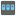• # AssertionError

## Question related to missionHouse Password

def is_ascii(s): return all(ord(c) < 128 for c in s)

def checkio(pw): if is_ascii(pw) == True: if 9 < len(pw) <= 64: upper = 0 lower = 0 num = 0

```        for c in pw:
if c.isupper():
upper += 1
elif c.islower():
lower += 1
elif c.isdigit():
num += 1

if upper != 0 and lower != 0 and num != 0:
print(True)

else:
print(False)

else:
print(False)
else:
print(False)
```

above is my code, I checked with checkio("ULFFunH8ni") with pycharm, everything are correct, but it shows fail when i submit it5